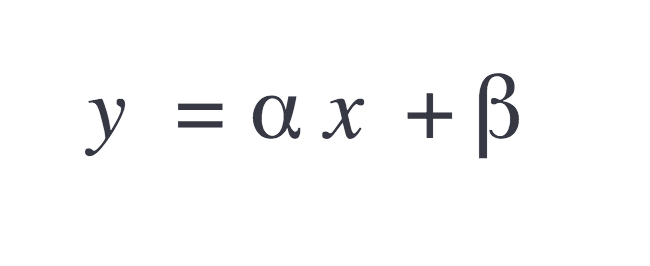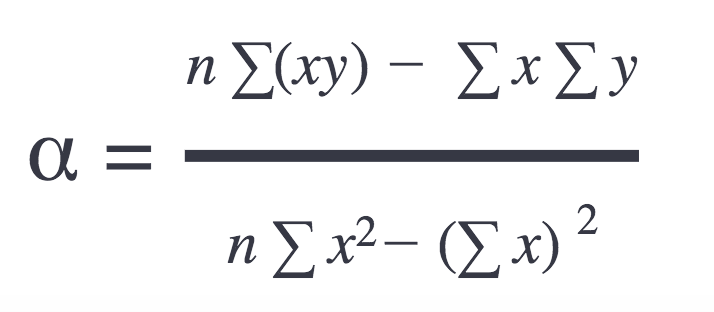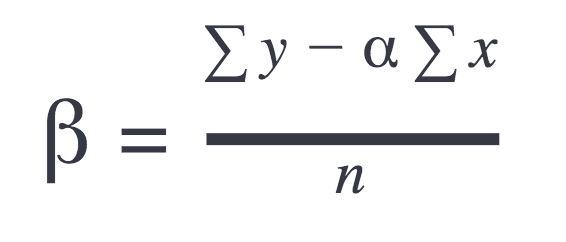# Custom technical indicators

Be sure to have at least the Highcharts version 6, as previous Highcharts versions don’t support technical indicators.

## TECHNICAL INDICATOR SERIES

There are two main steps to create a technical indicator series:

1. Set up the technical indicator structure.
2. Create the technical indicator functionality.

### 1. Set up the structure

Each technical indicator requires the method `getValues()` to be implemented. This method takes two arguments and returns an object. The arguments are the main series and the parameters. The parameters are specific to a technical indicator. Check the structure of the method `getValues()`:

`function getValues(series, params) {    // calculations    ...    // end of calculations    return {      xData: [...], // array of x-values      yData: [...] // array of y-values      values: [...], // array of points    };  }`

All technical indicators are series types, and to create a new series, in Highcharts, the following method Highcharts.seriesType() is used.

`<script type="text/javascript" src="https://code.highcharts.com/stock/indicators/indicators.js"></script>Highcharts.seriesType(  'linearregression',  'sma',  {    name: 'Linear Regression',    params: {} // linear regression doesn’t need params  },  {    getValues: function (series, params) {      return this.getLinearRegression(series.xData, series.yData);    },    getLinearRegression: getLinearRegression  });`

The method `getLinearRegression()` includes the technical indicator functionality (mathematical calculation). Notice that the indicators module `indicators.js` is included when creating technical indicators, as it includes the core-logic for all indicators.

Now the structure is set, the next step is to create the main indicator functionality.

### 2. Technical indicator functionality

The technical indicator functionality is represented by the following method `getLinearRegression()`, that calculates the regression points according to xData and yData.

Here is a simple mathematical representation of the linear regression:Where the slope is:And offset:The JavaScript representation of the formulas above is as follows:

`function getLinearRegression(xData, yData) {  var sumX = (xValLength - 1) * xValLength / 2,      sumY = 0,      sumXY = 0,      sumX2 = ((xValLength - 1) * (xValLength) * (2 * xValLength - 1)) / 6,      linearData = [],      linearXData = [],      linearYData = [],      n = xData.length,      alpha, beta, i, x, y;  // Get sums:  for (i = 0; i < n; i++) {    y = yData[i];    sumY += y;    sumXY += i * y;  }  // Get slope and offset:  alpha = (n * sumXY - sumX * sumY) / (n * sumX2 - sumX * sumX);  if (isNaN(alpha)) {    alpha = 0;  }  beta = (sumY - alpha * sumX)/ n;  // Calculate linear regression:  for (i = 0; i < n; i++) {    y = alpha * i + beta;    // Prepare arrays required for getValues() method    linearData[i] = [xData[i], y];    linearXData[i] = xData[i];    linearYData[i] = y;  }  return {    xData: linearXData,    yData: linearYData,    values: linearData  };}`

Notice that Linear regression series in this example is still a line series, and that means data has to be sorted ascending by x-values.

That’s it; the technical indicator is ready to be used. Keep in mind that the technical indicator is connected to the main series by the linkedTo option:

`series: [{  id: 'main',  type: 'scatter',  data: [ ... ]}, {  type: 'linearregression',  linkedTo: 'main'}]`

For live demos check the links below:

Remark

To improve the user experience when using the linear regression series, try to disable tooltip and/or markers. Go to the `seriesType()` and set the default options as follows:

`Highcharts.seriesType(  'linearregression',  'sma',  {    name: 'Linear Regression',    enableMouseTracking: false, // default options    marker: {      enabled: false    }    params: {} // linear regression doesn’t need params  },  {    getValues: ... ,    getLinearRegression: ...  });`

Module The indicator is available as a indicators/trendline.js main module.

### 3. Indicator with multiple lines

Highcharts Stock offers many already implemented ready-to-use indicators. Some of them are drawn using multiple lines (e.g. Bollinger Bands). In this section, you will learn how to build a custom indicator consisting of 5 lines.

Let's assume we would like to build an indicator with a linear regression main line from the previous example, but this time with the four additional range lines that help to visualize the difference between the main series line and our regression line. For that, we'll add 4 additional lines that will be treated as zones. Those lines' values will be multiplied accordingly by 90%, 95%, 105%, and 110% of the regression line values (step ratio would be possible to change in `series.params`).

Of course, the data from the previous example has to be modified:

`// ...var zoneDistance = this.options.params.zoneDistance / 100;// ...// Calculate linear regression:for (i = 0; i < n; i++) {  x = xData[i];  y = alpha * x + beta;  // Prepare arrays required for getValues() method  linearData[i] = [x, y * (1 - 2 * zoneDistance), y * (1 - zoneDistance), y, y * (1 + zoneDistance), y * (1 + 2 * zoneDistance)];  linearXData[i] = x;  linearYData[i] = [y * (1 - 2 * zoneDistance), y * (1 - zoneDistance), y, y * (1 + zoneDistance), y * (1 + 2 * zoneDistance)];}return {  xData: linearXData,  yData: linearYData,  values: linearData};`

To draw an indicator with multiple lines, we can use the Highcharts MultipleLines mixin that can be accessed after we add any of the Highcharts indicators implementing multiple lines, e.g. Bollinger Bands:

`<script type="text/javascript" src="https://code.highcharts.com/stock/indicators/bollinger-bands.js"></script>`

Now, when having access to the MultipleLines mixin, we can prepare Highcharts to draw multiple lines by overwriting the default series prototype methods. We can also name each additional line and define its own styles. Inside the `Highcharts.seriesType` method we can define the following properties (* - required):

• `params`* - indicator's parameters. In our case, we set the default difference between the lines to `5%`. This can be changed in the indicator's series options.
• `closeRangeBottomLine`, `highRangeBottomLine`, `closeRangeTopLine`, `highRangeTopLine` - separate default styles for each additional line. This can be changed in the indicator's series options.
• `linesApiNames`* - an array containing the names of the additional lines.
• `nameBase` - the name of the indicator displayed in the legend.
• `nameComponents` - an array containing the names of the properties that values should be displayed in parenthesis in the legend next to the indicator's name.
• `pointArrayMap`* - an array containing the keys of the points' values.
• `pointValKey`* - default value for pointValKey
• `drawGraph`, `getTranslatedLinesNames`, `translate`, `toYData` - overwrite the Highcharts core methods responsible for drawing multiple lines.

Here is how the seriesType method's arguments should look like:

`var multipleLinesMixin = Highcharts._modules['Mixins/MultipleLines.js'];Highcharts.seriesType(  'linearregressionzones',  'sma',  {    color: '#00ff00',    params: {      zoneDistance: 5    },    tooltip: {      pointFormat: '<span style="color:{point.color}">\u25CF</span> {series.name}:<br/>' +        '110%: <b>{point.y4}</b><br/>' +        '105%: <b>{point.y3}</b><br/>' +        '100%: <b>{point.y}</b><br/>' +        '95%: <b>{point.y2}</b><br/>' +        '90%: <b>{point.y1}</b>'    },    closeRangeBottomLine: {      styles: {        lineWidth: 1,        lineColor: '#ffa500'      }    },    highRangeBottomLine: {      styles: {        lineWidth: 1,        lineColor: '#ff0000'      }    },    closeRangeTopLine: {      styles: {        lineWidth: 1,        lineColor: '#ffa500'      }    },    highRangeTopLine: {      styles: {        lineWidth: 1,        lineColor: '#ff0000'      }    }  },  {    getValues: function (series) {        return this.getLinearRegressionZones(series.xData, series.yData);    },    getLinearRegressionZones: getLinearRegressionZones,    linesApiNames: ['highRangeBottomLine', 'closeRangeBottomLine', 'closeRangeTopLine', 'highRangeTopLine'],    nameBase: 'Linear regression zones',    nameComponents: ['zoneDistance'],    nameSuffixes: ['%'],    parallelArrays: ['x', 'y', 'y1', 'y2', 'y3', 'y4'],    pointArrayMap: ['y1', 'y2', 'y', 'y3', 'y4'],    pointValKey: 'y'  });var multipleLinesMixin = Highcharts._modules['Mixins/MultipleLines.js'];if (multipleLinesMixin) {  Highcharts.extend(    Highcharts.seriesTypes.linearregressionzones.prototype,    {      drawGraph: multipleLinesMixin.drawGraph,      getTranslatedLinesNames: multipleLinesMixin.getTranslatedLinesNames,      translate: multipleLinesMixin.translate,      toYData: multipleLinesMixin.toYData    }  );} else { // Highcharts v9.2.3+  Highcharts._modules['Stock/Indicators/MultipleLinesComposition.js'].compose(    Highcharts.seriesTypes.linearregressionzones  );}`

A live demo of the above multiline indicator can be found here.

For more detailed samples and documentation check the API.

### 4. Indicators calculated on events.

The idea behind creating a custom indicator that is calculated after some chart event (for example `afterSetExtremes`) is similar to the ones previously mentioned with a few additions.

The most important object to add while creating the indicators is `calculateOn` where inside are specified two properties:

• `chart` - on which chart's event the indicator should be calculated, mainly you can choose from `init` (before the linked series is processed) and `render` (after processing the linked series).
• `xAxis` - on which xAxis' event the indicator should be recalculated (e.g. `afterSetExtremes`),

Example configuration should look like:

`Highcharts.seriesType('customIndicator', 'sma', {}, {    getValues: function (series) {        return this.getSum(            series.processedXData || series.xData,            series.processedYData || series.yData        );    },    calculateOn: {        chart: 'init',        xAxis: 'afterSetExtremes'    },    getSum: getSum});`

A live demo of the example above can be found here.

### 5. Custom column indicator.

The principle of creating a custom column indicator is similar to the ordinary one. Still, the `getValues` method for calculating points position is required. Along with that, in order to render the column, some other methods like `crispCol`, `drawPoints`, `getColumnMetrics`, `translate` need to be inherited from the column series prototype and the `markerAttribs`, `drawGraph` need to be overwritten with an empty function as shown in the code snippet below.

Additionally, to correctly render that indicator, the `threshold`, `groupPadding` and `pointPadding` properties need to be defined.

Example configuration should look like:

`Highcharts.seriesType(    'customIndicator',    'sma', {        name: 'Sum of previous 2 points',        threshold: 0,        groupPadding: 0.2,        pointPadding: 0.2    }, {        getValues: function(series) {            return this.getSum(series.xData, series.yData);        },        getSum: getSum,        markerAttribs: Highcharts.noop,        drawGraph: Highcharts.noop,        crispCol: Highcharts.seriesTypes.column.prototype.crispCol,        drawPoints: Highcharts.seriesTypes.column.prototype.drawPoints,        getColumnMetrics: Highcharts.seriesTypes.column.prototype.getColumnMetrics,        translate: Highcharts.seriesTypes.column.prototype.translate    });`

A live demo of the example above can be found here.